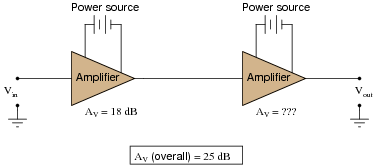# Decibel Measurements

## AC Electric Circuits

• #### Question 1

During the early development of telephone technology, a unit was invented for representing power gain (or loss) in an electrical system. It was called the Bel, in honor of Alexander Graham Bell, the telecommunications pioneer.

“Bels” relate to power gain ratios by the following equation:

 AP(ratio) = 10AP(Bels)

Given this mathematical relationship, translate these power gain figures given in units of Bels, into ratios:

AP = 3 B ; AP =
AP = 2 B ; AP =
AP = 1 B ; AP =
AP = 0 B ; AP =
AP = -1 B ; AP =
AP = -2 B ; AP =
AP = -3 B ; AP =
• #### Question 2

Manipulate this equation algebraically, so that we can convert power gains expressed in units of Bels, into ratios.

 AP(ratio) = 10AP(Bels)

Then, convert the following power gains, expressed as ratios, into units of Bels:

AP = 250 ; AP =
AP = 1275 ; AP =
AP = 10 ; AP =
AP = 1 ; AP =
AP = 0.1 ; AP =
AP = 0.025 ; AP =
AP = 0.00009 ; AP =
• #### Question 3

At some point in time, it was decided that the unit of the “Bel” was too large. Instead, the deci-Bel became the most common usage of the unit. Modify these equations to include AP figures cast in units of decibels (dB) instead of Bels:

 AP(ratio) = 10AP(Bels)

 AP(Bels) = logAP(ratio)

Then, calculate the decibel figures that correspond to a power gain of 2 (ratio), and a power loss of 50%, respectively.

• #### Question 4

Suppose an AC signal amplifier circuit has a voltage gain (ratio) of 2. That is, Vout is twice as large as Vin: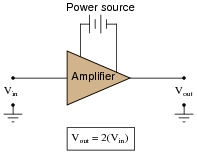• #### Question 5

Suppose an AC signal amplifier circuit has a voltage gain (ratio) of 2. That is, Vout is twice as large as Vin: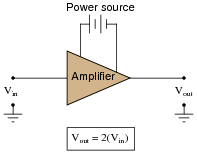If we were to try to rate this amplifier’s gain in terms of the relative power dissipated by a given load resistance (Pload when powered by Vout, versus Pload when powered by Vin), what decibel figure would we calculate?

• #### Question 6

Voltage and current gains, expressed in units of decibels, may be calculated as such:

 AV(dB) = 10 log( AV(ratio) ) 2

 AI(dB) = 10 log( AI(ratio) ) 2

Another way of writing this equation is like this:

 AV(dB) = 20 logAV(ratio)

 AI(dB) = 20 logAI(ratio)

What law of algebra allows us to simplify a logarithmic equation in this manner?

• #### Question 7

Convert the following amplifier gains (either power, voltage, or current gain ratios) into gains expressed in the unit of decibels (dB):

AP = 25 ; AP(dB) =
AV = 10 ; AV(dB) =
AI = 37 ; AI(dB) =
AP = 150 ; AP(dB) =
AI = 41 ; AI(dB) =
AV = 3.4 ; AV(dB) =
AP = 18 ; AP(dB) =
AV = 100 ; AV(dB) =
• #### Question 8

Convert the following amplifier gains expressed in the unit of decibels (dB), to gain figures expressed as unitless ratios:

AP = 5 dB ; AP(ratio) =
AV = 23 dB ; AV(ratio) =
AI = 20 dB ; AI(ratio) =
AP = 2.5 dB ; AP(ratio) =
AI = 7.4 dB ; AI(ratio) =
AV = 45 dB ; AV(ratio) =
AP = 12.8 dB ; AP(ratio) =
AV = 30 dB ; AV(ratio) =
• #### Question 9

Convert the following amplifier gains between decibels and (unitless) ratios as necessary:

AV = 14.1 dB ; AV(ratio) =
AI = 202 ; AI(dB) =
AP = 15 dB ; AP(ratio) =
AI = 33 ; AI(dB) =
AP = 49 dB ; AP(ratio) =
AV = 57 ; AV(dB) =
AP = 8.8 dB ; AP(ratio) =
AV = 30 ; AV(dB) =
• #### Question 10

What does it mean to speak of the gain of a circuit? This term is very commonly used when describing amplifier circuits, but it may also be used to describe circuits containing nothing but passive components, and are thus incapable of amplifying.

What letter is used to symbolize gain in mathematical equations?

• #### Question 11

Calculate the voltage gain of this circuit, if R1 has a resistance of 8.1 kΩ and R2 has a resistance of 1.75 kΩ: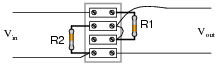• #### Question 12

Calculate the power gain of this circuit, if R1 has a resistance of 1 kΩ, R2 has a resistance of 5.1 kΩ, and the load has a resistance of 10 kΩ: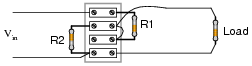• #### Question 13

Suppose an AC signal amplifier circuit has a voltage gain (ratio) of 5. That is, Vout is five times as large as Vin: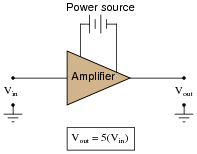Translate this voltage gain ratio into a decibel figure. Explain why the conversion from voltage gain ratio to decibels is not the same as conversion of a power gain ratio to decibels.

• #### Question 14

Specialized forms of the decibel unit have been devised to allow easy representation of quantities other than arbitrary ratios of voltage, current, or power. Take for example these units, the first one used extensively in the telecommunications industry:

dBm
dBW
dBk

Define what each of these units represents.

• #### Question 15

Calculate the overall voltage gain of this cascaded amplifier circuit, where the output of one voltage amplifier feeds into the input of another: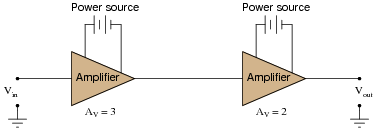Also, convert the voltage gains of each amplifier into units of decibels, then convert the overall voltage gain ratio into units of decibels as well.

What do you notice about the overall gain of this circuit in relation to the individual amplifier gains, compared as ratios versus compared as decibel figures?

• #### Question 16

Calculate the overall voltage gain of this cascaded amplifier circuit, where the output of one voltage amplifier feeds into the input of another: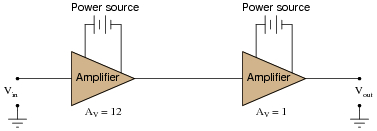Also, convert the voltage gains of each amplifier into units of decibels, then convert the overall voltage gain ratio into units of decibels as well.

What do you notice about the overall gain of this circuit in relation to the individual amplifier gains, compared as ratios versus compared as decibel figures?

• #### Question 17

In this cascaded voltage divider circuit, determine the overall voltage gain ratio (from first input to last output), and also calculate the overall voltage gain in decibels, as well as the decibel figure for each divider’s voltage gain:What do you notice about the ratio figures versus the decibel figures, regarding how the individual stage gains compare with the overall gain?

• #### Question 18

In this circuit, one amplifier feeds into an attenuator circuit, which then feeds into a second amplifier stage. Calculate the “gain” of the attenuator, and then calculate the overall voltage gain of this three-stage circuit: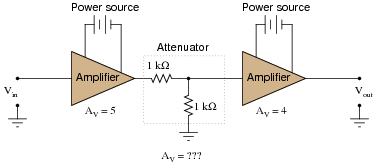Also, convert the voltage gains of each stage into units of decibels, then convert the overall voltage gain ratio into units of decibels as well.

What do you notice about the overall gain of this circuit in relation to the individual amplifier gains, compared as ratios versus compared as decibel figures?

• #### Question 19

Calculate the necessary gain of the second-stage amplifier to give the whole circuit a voltage gain of 25 decibels, then translate all decibel figures into gain ratios: